# Chemical Kinetics Chemistry Class 12 Notes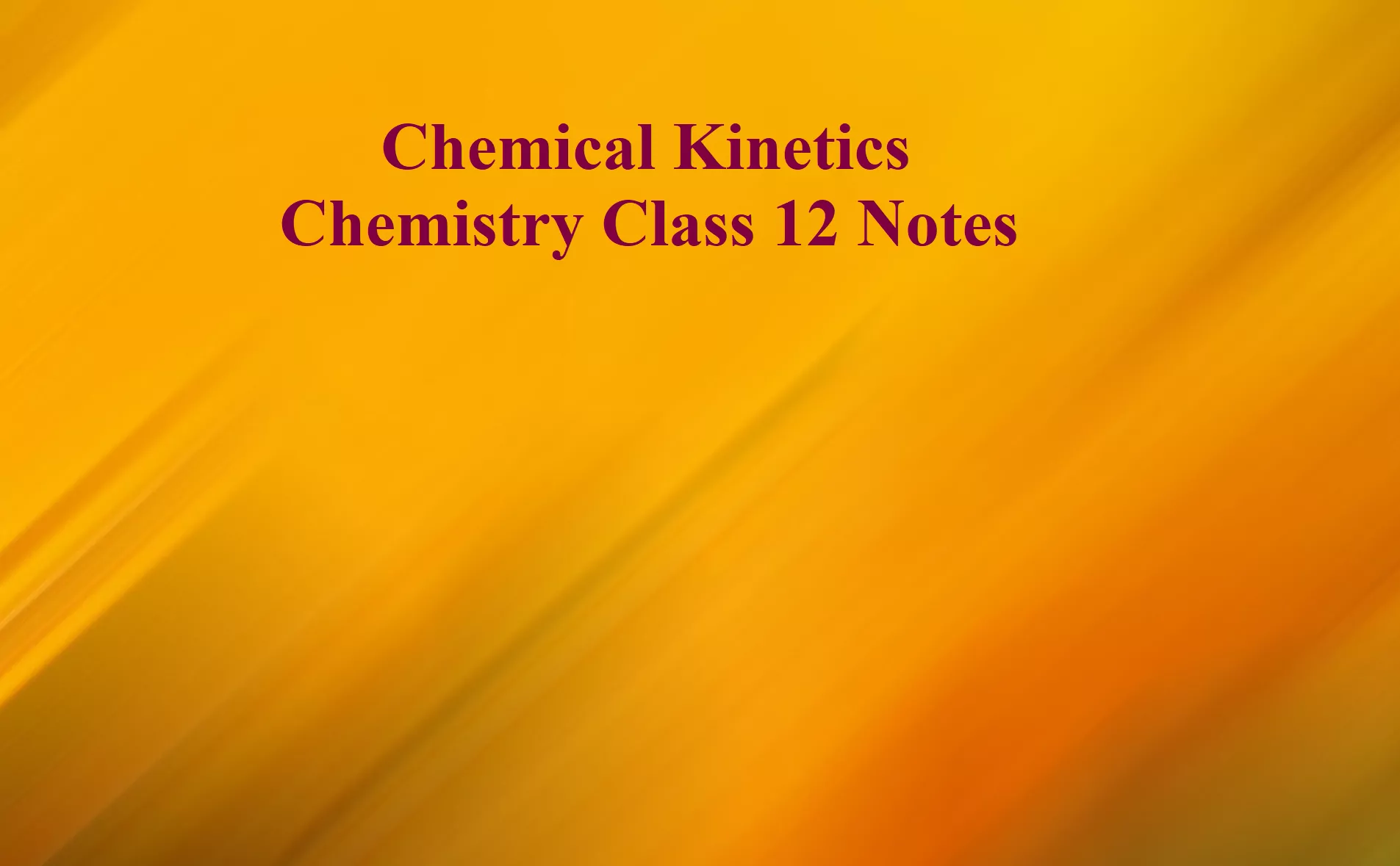Chemical Kinetics Chemistry Class 12 Notes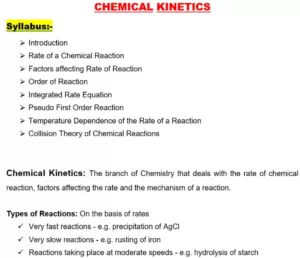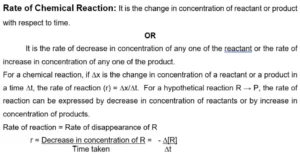-ve sign before ΔR indicates the decrease in the concentration of reactant.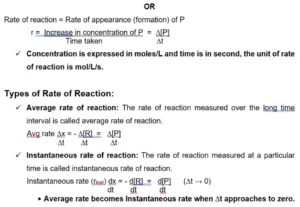Chemical Kinetics Chemistry Class 12 Notes

Factors Affecting Rate of Reaction:
i). The concentration of reactant
ii). Surface area
iii). Temperature
iv). Nature of reactant
v). Presence of catalyst.

Dependence rate on Concentration:
Rate Law: The rate of reaction is directly proportional to the product of the concentration of reactant and each concentration is raised to some power which may or may not be equal to stereochemistry experimentally.
For a general reaction, aA + bB → cC + dD,
Rate α [A]x[B]y
Rate = k[A]x[B]y (Where x and y may or may not equal to a & b).
k is proportionality constant and is called Rate Constant
Rate Constant (k): Rate constant is a rate of reaction when molar concentration of reactant is unity.

Chemical Kinetics Chemistry Class 12 Notes

Molecularity of a Reaction: The no. of molecules of the reactants involved in the reaction is known as Molecularity. It cannot be zero or fractional. It can have values 1,2,3 etc. it is applicable only to elementary reactions.
If the molecularity of a reaction is 1, it is called Unimolecular Reaction. e.g. Decomposition of ammonium nitrite NH4NO2 → N2 + 2 H2O.
If the molecularity of a reaction is 2, it is called a Bimolecular Reaction. e.g. Decomposition of Hydrogen Iodide   2 HI → H2 + I2
Order of Reaction: Order is the sum of the powers of the concentration terms of the reactants in the rate law. It is an experimental quantity. It can have the values 0,1,2,3… or a fraction. It is applicable to both elementary and complex reactions.
For a general reaction, aA + bB → cC + dD
der of a reaction is zero, it is called Zero Order Reaction, if it is one, it is called First Order Reaction, if it is two, it is called Second Order Reaction, and so on.

 S.No. Order Molecularity 1. It is the sum of the powers of the concentration terms in the rate law expression. It is the total number of reactant species that collide simultaneously in a chemical reaction. 2. It is an experimental quantity. It is a theoretical quantity. 3. It can be zero or fractional. It cannot be zero or fractional. 4. It is applicable to both elementary and complex reactions. It is applicable only to elementary reactions.

Units of Rate Constant
Different ordered reactions have different units for k.
For an nth order reaction
For the reaction, nA → Products
rate =  k[A]n
Therefore, k = rate                  …………………. (1)
[Concentration]n
From relation (1) we can find order for various reactions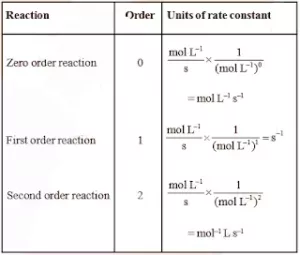Chemical Kinetics Chemistry Class 12 Notes

Integrated Rate Equations: These are equations relating to the rate of a reaction and concentration of reactants. Different ordered reactions have different integrated rate law equations.
1). For Zero Order Reaction: Zero-order reactions are reactions in which the rate of reaction is proportional to zero power of the concentration of reactants.
Consider a zero-order reaction     R → P
The rate expression for the above reaction is
r = – d[R] ………………. (1)
dt
Rate law for the above reaction is
r= k[R]0 = k ………………… (2)
From equations (1) & (2), we can write
k  =   – d[R]
dt
The above equation is known as the differential rate equation for the zero-order reaction.
d[R] = – kdt
On integrating the above equation, we get
[R] = – kt + C      …………………. (3)
Where C is the constant of integration. To calculate the value of C, consider the initial conditions. i.e., when t=0, [R] = [R]0
titute these values in equation (3)
[R]0 = – k × 0 + C
C = – [R]0
Substituting C in equation (3), we get
[R] = – kt  –  [R]0  …………. (4)
[R]0 – [R] = kt

 k = [R]0 – [R]              t

………………. (5)

This equation is of the form of a straight line y = mx + c. So if we plot [R] against t, we get a straight line with slope = – k and intercept equal to [R]0.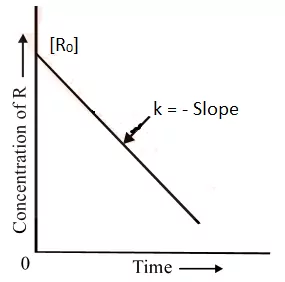The decomposition of gaseous ammonia on a hot platinum surface at high pressure.
N2 + H2 → 2 NH3
At high pressure, molecules. So, a further change in reaction conditions does not change the rate of the reaction. So, it becomes a zero-order reaction.
Another e.g. is the thermal decomposition of HI on gold surface.

Chemical Kinetics Chemistry Class 12 Notes

2. For First Order Reaction: First order reactions are reactions in which the rate of reaction is proportional to the first power of the concentration of reactants.
Consider a first-order reaction     R → P
The rate expression for the above reaction is
r = – d[R] ………………. (1)
dt
Rate law for the above reaction is
r= k[R]1 = k ………………… (2)
From equations (1) & (2), we can write
k [R] = – d[R]
dt
d[R]   = – k dt
[R]
On integrating the above equation, we get
In [R] = – kt + C …….. (3)
Where C is the constant of integration. To calculate the value of C, consider the initial conditions. i.e., when t=0, [R] = [R]0
Substitute these values in equation (3)
In [R0] = – k × 0 + C
C = ln [R0]
Substituting C in equation (3), we get
ln[R] = – kt + ln[R0] ………………………………… (4)
Rearranging above equation we get
kt = ln[R0] – ln[R]

 k =   1  ln [R0]          t      [R]

 k =2.303 log[R0]          t          [R]

………… (A)

At time t1 from equation (4)
In [R1] = – kt1 + ln [R2]
At time t2
[R1] = – kt2 + ln [R2]
where, [R1] and [R2] are the concentrations of the reactants at time t1 and t2 respectively.
Subtracting
ln [R1] – ln [R2] = – kt1 – (–kt2)
ln [R1] = k (t2 – t1)
[R2]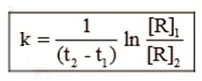Comparing equation (2) with y = mx + c, if we plot In [R] against t, we get a straight line with slope = – k and intercept equal to ln [R0].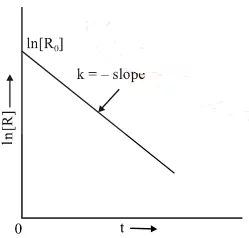The first-order rate equation (A) can also be written in the form
Log [R0] =     kt
[R]       2.303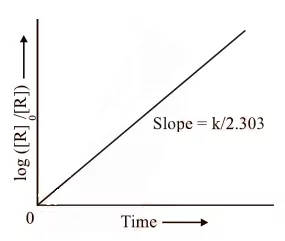For Example:
Hydrogenation of ethene:  C2H4(g) + H2(g) → C2H6(g);     r = k[C2H4]
All natural and artificial radioactive decay.

Chemical Kinetics Chemistry Class 12 Notes

Pseudo First Order Reaction: The reactions which are not truly of first order but become reactions of first-order under certain conditions are called pseudo-first-order reactions.
Let us take a general reaction
A + B → C
The rate of reaction depends upon the concentrations of both A and B but one of the components is present in large excess and thus its concentration hardly changes as the reaction proceeds so the rate is independent of that particular component.
So, if component B is in large excess and the concentration of B is very high as compared to that of A, the reaction is considered to be a pseudo-first-order reaction with respect to A and if component A is in large excess and the concentration of A is very high as compared to that of B, the reaction is considered to be pseudo-first order with respect to B.

For example,
i) Hydrolysis of ester in acid (H+).
CH3COOC2H5 + H2O → CH3COOH + C2H5OH
Rate = k[CH3COOC2H5] [H2O]
Since water is in excess so its concentrations remain the same during the reaction and [H2O] is constant and the rate is independent of the concentration of H2O so
Rate = k[CH3COOC2H5], Where k = k[H2O]

ii) Inversion of cane sugar (in acid)
C12H22O11 + H2O → C6H12O6   +    C6H12O6
Cane sugar                   Glucose           Fructose
Since water is in excess and [H2O] is constant, so
Rate = k [C12H22O11]

(In the above reactions the concentration of H2O is in excess so the rate of reaction is independent of the concentration of water).

Chemical Kinetics Chemistry Class 12 Notes

The half-life of a reaction (t1/2): It is the time in which the concentration of a reactant is reduced to one-half of its initial concentration. It is represented by t1/2.
(i) Half-Life of a Zero Order Reaction: For a zero-order reaction, the integrated rate law is:
k = [R0] – [R]
t
When t = t1/2, [R] = ½ [R0]
On substituting these values in the above equation,
k = [R0] – ½ [R0]
t1/2

 t1/2 = [R0]           2k

From the above relation, we can say Half-life of a zero-order reaction is directly proportional to the initial concentration of the reactants and inversely proportional to the rate constant.

(ii) Half-Life of a First Order Reaction: For a first-order reaction, the integrated rate law is: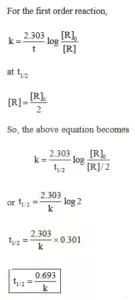Rate of Reaction and Temperature: Most of the chemical reactions are accelerated by the increase in temperature. It has been found that for a chemical reaction when the temperature is increased by 10°, the rate of the reaction and the rate constant is nearly doubled.  The ratio of the rate constants of a reaction at two temperatures differing by 100 is called the temperature coefficient.
i.e., Temperature coefficient = Rate constant of the reaction at (T + 10)K
The rate constant of the reaction at T K
The temperature dependence of the rate of a chemical reaction can be accurately explained by the Arrhenius equation.  The equation is:
k = A e – Ea /RT ……………………………….. (1)

Where A is a constant called the Arrhenius parameter or the frequency factor or the pre-exponential factor. It is constant for a particular reaction.
R is the universal gas constant and Ea is activation energy measured in joules/mole (J mol –1).

Chemical Kinetics Chemistry Class 12 Notes

Threshold Energy: The minimum energy which the colliding molecules must have in order that the collision between them may be effective is called threshold energy.

Activation Energy: The minimum extra amount of energy absorbed by the reactant molecules so that their energy becomes equal to the threshold value is called activation energy.
Threshold energy = Activation energy + Energy possessed by the reactants
Less is the activation energy, faster is the reaction. In order that the reactants may change into products, they have to cross an energy barrier (corresponding to threshold
energy). Reactant molecules absorb energy and form an intermediate called activated complex which immediately dissociates to form the products.
For Example: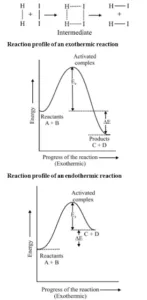Chemical Kinetics Chemistry Class 12 Notes

Arrhenius equation:
Quantitatively, the temperature dependence of the rate of a chemical reaction can be explained by Arrhenius equation
k = A e – Ea /RT
where A is the Arrhenius factor or the frequency factor or the pre-exponential factor. R is gas constant and E a is activation energy measured in joules/mole.
The factor e – Ea /RT corresponds to the fraction of molecules that have kinetic energy greater than Ea.
Thus, it has been found from the Arrhenius equation that increasing the temperature or decreasing the activation energy will result in an increase in the rate of the reaction and an exponential increase in the rate constant.
Taking the natural logarithm of both sides of the equation
ln K = – E + ln A
RT
At temperature T1, equation
ln K1 =  – E + ln A
RT1
At temperature T2, equation
ln K2 = –  E + ln A
RT2
(since A is constant for a given reaction)
K1 and K2  are the values of rate constants at temperatures T1 and T2 respectively.
Subtracting equation form, we obtain
ln K2 – ln K1 =    E  –   E
RT1     RT2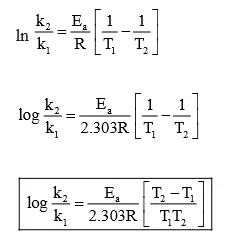Effect of Catalyst: A catalyst is a substance that alters the rate of a reaction without itself undergoing any permanent chemical change. The action of the catalyst can be explained by intermediate complex theory. According to this theory, a catalyst participates in a chemical reaction by forming an intermediate complex. This is unstable and decomposes to yield products and the catalyst.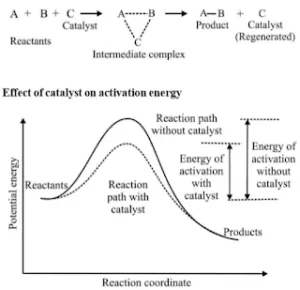A catalyst increases the rate of a chemical reaction by providing an alternate pathway or reaction mechanism by reducing the activation energy between reactants and products. The important characteristics of a catalyst are:

1. A small amount of the catalyst can catalyze a large number of reactants.
2. A catalyst does not alter Gibb’s energy, ΔG of a reaction. It catalyzes spontaneous reactions but does not catalyze non-spontaneous reactions.
3. A catalyst does not change the equilibrium constant of a reaction, but it helps to attain the equilibrium faster by increasing the rate of both forward as well as backward reactions.

Collision Theory: This theory was developed by Max Trautz and William Lewis. It is based on the kinetic theory of gases. According to this theory, the reactant molecules are assumed to be hard spheres, and reaction has occurred when molecules collide with each other. The number of collisions per second per unit volume of the reaction mixture is known as collision frequency (Z).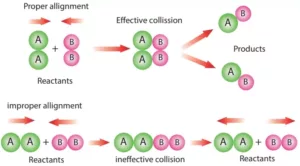Another factor that affects the rate of chemical reactions is the activation energy.
For a bimolecular elementary reaction
A + B → Products
The rate of reaction can be expressed as
Rate (r)= ZAB e – Ea /RT
Where ZAB represents the collision frequency of reactants, A and B, and e– Ea/RT represents the fraction of molecules with energies equal to or greater than EaComparing with the Arrhenius equation, we can see that A is related to collision frequency.
A third factor that affects the rate of a chemical reaction is the proper orientation. To account for this, a factor P called the Probability or Steric factor is introduced. So the above equation becomes:

Rate (r)= PZAB e – Ea /RT

Chemical Kinetics Chemistry Class 12 Notes